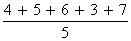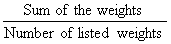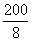## Definition Of Mean

Mean of a set of numbers is the sum of the numbers divided by the number of items in the list. Mean of a set of n numbers a1, a2, a3, ..., an is given byMean can also be called as average or arithmetic mean.

### Examples of Mean

In order to find the mean of 4, 5, 6, 3, and 7, first we have to add the numbers and then divide the sum by the number of items.
4 + 5 + 6 + 3 + 7 = 25 i.e. the sum of the numbers is 25.
Mean ==== 5
So, the mean of the data set 4, 5, 6, 3, and 7 is 5.

### Solved Example on Mean

#### Ques: Find the mean weight of the data set shown. 5 lb, 48 lb, 31 lb, 31 lb, 41 lb, 20 lb, 19 lb, 5 lb

##### Choices:

A. 27 lb
B. 25 lb
C. 26 lb
D. 24 lb
Step 1: Mean weight =Step 5: Mean weight == 25 lb [Substitute and simplify.]